The OpenFOAM Foundation
rigidBodyInertia Class Reference

This class represents the linear and angular inertia of a rigid body by the mass, centre of mass and moment of inertia tensor about the centre of mass. More...

Inheritance diagram for rigidBodyInertia: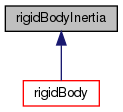[legend]

## Public Member Functions

rigidBodyInertia ()
Null constructor, initializes to zero. More...

rigidBodyInertia (const scalar m, const vector &c, const symmTensor &Ic)
Construct from mass, centre of mass and moment of inertia tensor. More...

rigidBodyInertia (const dictionary &dict)
Construct from dictionary. More...

rigidBodyInertia (const spatialTensor &st)
Construct from the components of a spatial tensor. More...

rigidBodyInertia (Istream &is)
Construct from Istream. More...

scalar m () const
Return the mass of the rigid-body. More...

const vectorc () const
Return the centre of mass of the rigid-body. More...

const symmTensorIc () const
Return the inertia tensor of the rigid-body about the centre of mass. More...

symmTensor Ioc () const
Return the difference between the inertia tensor of the rigid-body. More...

symmTensor Icc (const vector &c) const
Return the difference between the inertia tensor of the rigid-body. More...

symmTensor Io () const
Return the inertia tensor of the rigid-body about the origin. More...

scalar kineticEnergy (const spatialVector &v)
Return the kinetic energy of the body with the given velocity. More...

operator spatialTensor () const
Conversion to spatial tensor. More...

void operator+= (const rigidBodyInertia &)

## Static Public Member Functions

static symmTensor Ioc (const scalar m, const vector &c)
Return the difference between the inertia tensor of the rigid-body. More...

## Friends

Istreamoperator>> (Istream &, rigidBodyInertia &)

Ostreamoperator<< (Ostream &, const rigidBodyInertia &)

## Detailed Description

This class represents the linear and angular inertia of a rigid body by the mass, centre of mass and moment of inertia tensor about the centre of mass.

Reference:

```    Featherstone, R. (2008).
Rigid body dynamics algorithms.
Springer.
```
Source files

Definition at line 75 of file rigidBodyInertia.H.

## ◆ rigidBodyInertia() [1/5]

 rigidBodyInertia ( )
inline

Null constructor, initializes to zero.

Definition at line 44 of file rigidBodyInertiaI.H.

Referenced by cuboid::cuboid(), Foam::RBD::operator+(), sphere::sphere(), and Foam::RBD::transform().

Here is the caller graph for this function: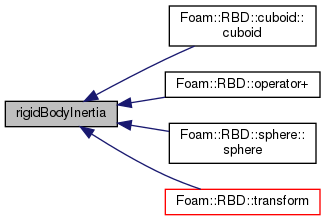## ◆ rigidBodyInertia() [2/5]

 rigidBodyInertia ( const scalar m, const vector & c, const symmTensor & Ic )
inline

Construct from mass, centre of mass and moment of inertia tensor.

about the centre of mass

Definition at line 53 of file rigidBodyInertiaI.H.

## ◆ rigidBodyInertia() [3/5]

 rigidBodyInertia ( const dictionary & dict )
inline

Construct from dictionary.

Definition at line 65 of file rigidBodyInertiaI.H.

## ◆ rigidBodyInertia() [4/5]

 rigidBodyInertia ( const spatialTensor & st )
inlineexplicit

Construct from the components of a spatial tensor.

Definition at line 73 of file rigidBodyInertiaI.H.

## ◆ rigidBodyInertia() [5/5]

 rigidBodyInertia ( Istream & is )
inlineexplicit

Construct from Istream.

Definition at line 81 of file rigidBodyInertiaI.H.

## ◆ Ioc() [1/2]

 Foam::symmTensor Ioc ( const scalar m, const vector & c )
inlinestatic

Return the difference between the inertia tensor of the rigid-body.

about the origin - about the centre of mass for the given mass and centre of mass

Definition at line 33 of file rigidBodyInertiaI.H.

References Foam::I, Foam::magSqr(), and Foam::sqr().

Here is the call graph for this function: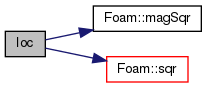## ◆ m()

 Foam::scalar m ( ) const
inline

Return the mass of the rigid-body.

Definition at line 91 of file rigidBodyInertiaI.H.

Here is the caller graph for this function: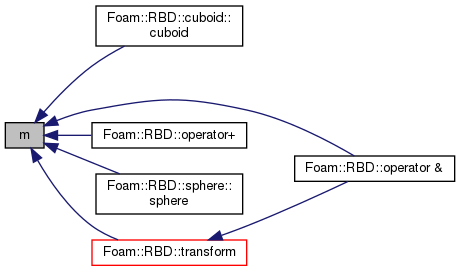## ◆ c()

 const Foam::vector & c ( ) const
inline

Return the centre of mass of the rigid-body.

Definition at line 96 of file rigidBodyInertiaI.H.

Here is the caller graph for this function: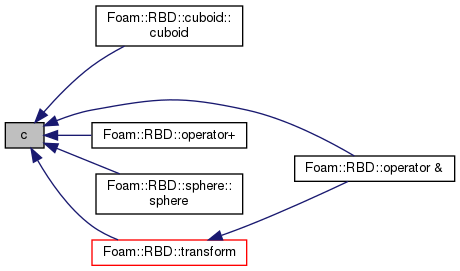## ◆ Ic()

 const Foam::symmTensor & Ic ( ) const
inline

Return the inertia tensor of the rigid-body about the centre of mass.

Definition at line 101 of file rigidBodyInertiaI.H.

Referenced by Foam::RBD::operator+(), and Foam::RBD::transform().

Here is the caller graph for this function: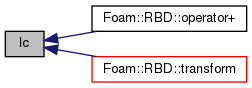## ◆ Ioc() [2/2]

 Foam::symmTensor Ioc ( ) const
inline

Return the difference between the inertia tensor of the rigid-body.

about the origin - about the centre of mass

Definition at line 106 of file rigidBodyInertiaI.H.

Referenced by rigidBodyInertia::Icc(), and rigidBodyInertia::Io().

Here is the caller graph for this function: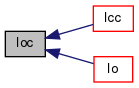## ◆ Icc()

 Foam::symmTensor Icc ( const vector & c ) const
inline

Return the difference between the inertia tensor of the rigid-body.

about the a new centre of mass - about the current centre of mass

Definition at line 111 of file rigidBodyInertiaI.H.

References rigidBodyInertia::Ioc().

Referenced by Foam::RBD::operator+().

Here is the call graph for this function: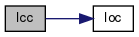Here is the caller graph for this function: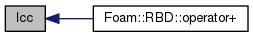## ◆ Io()

 Foam::symmTensor Io ( ) const
inline

Return the inertia tensor of the rigid-body about the origin.

Definition at line 116 of file rigidBodyInertiaI.H.

References rigidBodyInertia::Ioc().

Referenced by Foam::RBD::operator &(), and rigidBodyInertia::operator spatialTensor().

Here is the call graph for this function: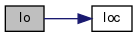Here is the caller graph for this function: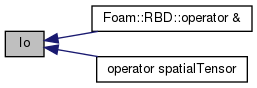## ◆ kineticEnergy()

 Foam::scalar kineticEnergy ( const spatialVector & v )
inline

Return the kinetic energy of the body with the given velocity.

Definition at line 233 of file rigidBodyInertiaI.H.

Referenced by Foam::RBD::transform().

Here is the caller graph for this function: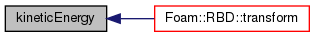## ◆ operator spatialTensor()

 operator spatialTensor ( ) const
inline

Conversion to spatial tensor.

Definition at line 124 of file rigidBodyInertiaI.H.

References Foam::I, and rigidBodyInertia::Io().

Here is the call graph for this function: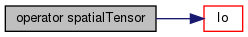## ◆ operator+=()

 void operator+= ( const rigidBodyInertia & rbi )
inline

Definition at line 244 of file rigidBodyInertiaI.H.

## ◆ operator>>

 Istream& operator>> ( Istream & , rigidBodyInertia & )
friend

## ◆ operator<<

 Ostream& operator<< ( Ostream & , const rigidBodyInertia & )
friend

The documentation for this class was generated from the following files: